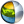Cg 3.1 Toolkit Documentation Cg / Standard Library / fwidth

## Name

fwidth - returns sum of approximate window-space partial derivatives magnitudes

## Synopsis

```float  fwidth(float a);
float1 fwidth(float1 a);
float2 fwidth(float2 a);
float3 fwidth(float3 a);
float4 fwidth(float4 a);

half   fwidth(half a);
half1  fwidth(half1 a);
half2  fwidth(half2 a);
half3  fwidth(half3 a);
half4  fwidth(half4 a);

fixed  fwidth(fixed a);
fixed1 fwidth(fixed1 a);
fixed2 fwidth(fixed2 a);
fixed3 fwidth(fixed3 a);
fixed4 fwidth(fixed4 a);
```

## Parameters

a
Vector or scalar of which to sum its approximate window-space partial derivative magnitudes. with respect to window-space X and Y.

## Description

Returns sum of the absolute values of each approximate partial derivative of a with respect to both the window-space (horizontal) x and (vertical) I<y) coordinate.

For vectors, the returned vector contains the sum of partial derivative magnitudes of each element of the input vector.

This function can be used to approximate the fragment width (hence the name "fwidth") for level-of-detail computations dependent on change in window-space.

This function is only available in fragment program profiles (but not all of them).

The specific way the partial derivative is computed is implementation-dependent. Typically fragments are rasterized in 2x2 arrangements of fragments (called quad-fragments) and the partial derivatives of a variable is computed by differencing with the adjacent horizontal fragment in the quad-fragment.

The partial derivative computation may incorrect when fwidth is used in control flow paths where not all the fragments within a quad-fragment have branched the same way.

The partial derivative computation may be less exact (wobbly) when the variable is computed based on varying parameters interpolated with centroid interpolation.

## Reference Implementation

fmod for float3 vectors could be implemented this way:

```float3 fwidth(float3 a)
{
return abs(ddx(a)) + abs(ddy(a));
}
```

## Profile Support

fwidth is supported only in fragment profiles. Vertex and geometry profiles lack the concept of window space.

fwidth is unsupported in the fp20, ps_1_1, ps_1_2, ps_1_3, and arbfp1 profiles.| Cg Toolkit | Download | Release Archive | Profiles | Reference | Books | Discussions |